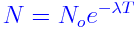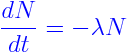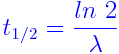The spontaneous breakdown of an atomic nucleus of a radioactive substance resulting in the emission of radiation from the nucleus is known as Radioactive decay. The nuclide which undergoes decay in a radioactive process is a parent nuclide, and the nuclide which is produced in the radioactive process is a daughter nuclide.

The Radioactive Formula is given byWhere N0 = the initial quantity of the substance and N is the quantity still remained and not yet decayed.

T is the half-life of the decaying quantity

e is the Euler’s number equal to 2.71828

The differential equation of Radioactive Decay Formula is defined asThe half-life of an isotope is the time taken by its nucleus to decay to half of its original number. It can be expressed asExample 1 – Carbon-14 has a half-life of 5.730 years. Determine the decay rate of Carbon-14.

Solution – If 100 mg of carbon-14 (N0 = 100) has a half- life of 5.730 years (t=5.730). The remaining 50 mg is present (N=50). We can use the formula.50 = 100 λ(5.730)

0.5 = e 5.730 λ

5.730 λ = ln 0.5

λ = ln0.5/5.730

λ = -0.525

Example 2 – 100 mg of neptunium -239(239Np) decays to 73.36mg in 24 hours. Determine the value of λ in growth decay formula for t in days?

Solution- Given that N = 73.36, N0 = 100 and t = 1(day), we can use the equation73.36 = 100 eλ(1)

0.7336 = eλ

λ = ln 0.7336

λ = -0.309791358

Therefore, the decay rate is approximately 31%.

To solve more problems on Radioactive formula and practice more questions, please visit Byjus.com